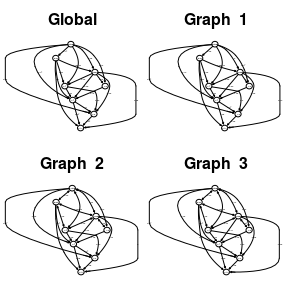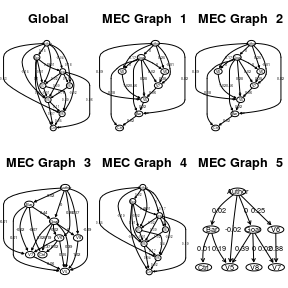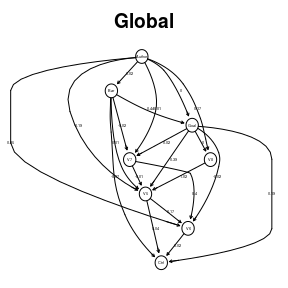IMaGES R Package and Algorithm

2018-12-14

Version 0.1

LazyData true

Repository CRAN

BugReports https://github.com/noahfl/IMaGES/issues

NeedsCompilation Yes

IMaGES Algorithm

This package is an implementation of the IMaGES algorithm of Ramsey, Hanson, Hanson, Halchenko, Poldrack, Glymour (2010) and is adapted from ‘GES’ as implemented in the ‘pcalg’ package. IMaGES (Independent Multi-sample Greedy Equivalence Search) is a score-based algorithm that greedily maximizes a score function similar to the one used in the pcalg implementation of GES. It modifies the scoring by creating a global score across all datasets and uses this score to determine which step from the individual datasets best represents all of the datasets. It accomplishes this by using forward, backward, and turning steps as described below:

• Forward phase In the forward phase, IMaGES moves through the space of essential graphs in steps that correspond to the addition of a single edge in the space of DAGs; the phase is aborted as soon as the score cannot be augmented any more.

• Backward phase In the backward phase, the algorithm performs moves that correspond to the removal of a single edge in the space of DAGs until the score cannot be augmented any more.

• Turning phase In the turning phase, the algorithm performs moves that correspond to the reversal of a single arrow in the space of DAGs until the score cannot be augmented any more.

During each step, the IMaGES algorithm simulates the addition, deletion, or turning of an edge for each individual dataset. The step that most augments the score (each edge is assigned a SEM BIC score) for the individual dataset is selected, and the global step across all datasets is selected by finding the most commonly recommended step. The algorithm then executes that step and updated the IMScore accordingly. This repeats for (number of nodes)**2 or until the algorithm detects that no steps augment the score for five consecutive iterations (also known as ‘early stopping’).

These operations are carried out and result in a global representative graph and a Markov equivalence class.

IMaGES in R

Description

Running this on the provided sample data returns an IMaGES object with a named list containing:

• .global a named list containing .graph, the global graphNEL object, and .params, the structural equation modeling data for the global graph

• .single.graphs a list containing named lists of the same structure as above that corresponds to each individual dataset passsed into IMaGES

• .markovs a list containing named lists of the same structure as above that corresponds to each Markov equivalence class (the size of which is specified by the user)

• .means a list containing the mean values for the structural equation modeling data for each edge in the graph

• .std.errs a list containing the standard errors for the structural equation modeling data for each edge in the graph

Usage

require(IMaGES)

data(IMData)

#run IMaGES
im.fits <- IMaGES(matrices=IMData, penalty=3, num.markovs=5, use.verbose=FALSE)
#>  "Running..."
#>  "Stopping early. IMaGES run has converged on a representative graph."
#>  "Done with IMaGES run."
#>  "Final IMScore:  220936.078265305"

plotAll

Description

This function takes the object returned by an IMaGES run and plots the global structure with its structural equation modeling data, as well as the structural equation modeling data for each dataset imposed on the global structure. The function determines the dimensions that most closely represent a square and plots the graphs in that fashion.

Usage

require(IMaGES)

data(IMData)

#run IMaGES
im.fits <- IMaGES(matrices=IMData, penalty=3, num.markovs=5, use.verbose=FALSE)
#>  "Running..."
#>  "Stopping early. IMaGES run has converged on a representative graph."
#>  "Done with IMaGES run."
#>  "Final IMScore:  220936.078265305"

#plot global graph and all individual graphs with own SEM data

par(mar=c(2,2,2,2))
plotAll(im.fits)plotMarkovs

Description

This function takes the object returned by an IMaGES run and plots the global structure with its structural equation modeling data, as well as the structural equation modeling data for each Markov equivalence class and their respective structures. The function determines the dimensions that most closely represent a square and plots the graphs in that fashion.

Usage

require(IMaGES)
data(IMData)

#run IMaGES
im.fits <- IMaGES(matrices=IMData, penalty=3, num.markovs=5, use.verbose=FALSE)
#>  "Running..."
#>  "Stopping early. IMaGES run has converged on a representative graph."
#>  "Done with IMaGES run."
#>  "Final IMScore:  220936.078265305"

#plot global graph alongside Markov equivalence class
plotMarkovs(im.fits)plotIMGraph

Description

This function takes a graph object returned from IMaGES (takes the form of a named list containing .graph and .params) and plots it. Using plotAll or plotMarkovs is recommended unless you only want to see one specific graph.

Usage

require(IMaGES)

plotIMGraph(im.fits\$.single.graph[])